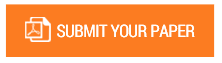Kharrat-Toma Transform and its Application in Solving Some Ordinary Differential Equations with Initial Boundary Conditions

Authors : MUSTAPHA R.A; SALAU A.M; BABATUNDE Idowu; OGABI C.O; IDOWU G.A

Volume/Issue : Volume 6 - 2021, Issue 8 - August

Scribd : https://bit.ly/3DhKnMi

In this paper, we adopted a new integral transform called Kharrat-Toma Transform which can be considered to be a basis for a number of potential new integral transforms. Some fundamental properties about this new integral transform were used in this work, includes the existence theorem, transportation theorem, convolution theorem and inversion equation. The major advantage of this new technique is that it solves ordinary differential equation with variable and constant coefficients. Some relevant examples were solved to show the efficiency of this technique.

Keywords : Kharrat-Toma Transform, Ordinary Differential Equations, Exact Solution

#### CALL FOR PAPERS

Paper Submission Last Date
31 - May - 2022